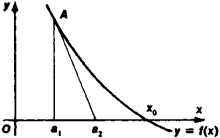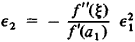# Newton's method

Also found in: Wikipedia.

## Newton's method

[′nüt·ənz ‚meth·əd]
(mathematics)
A technique to approximate the roots of an equation by the methods of the calculus.
McGraw-Hill Dictionary of Scientific & Technical Terms, 6E, Copyright © 2003 by The McGraw-Hill Companies, Inc.
The following article is from The Great Soviet Encyclopedia (1979). It might be outdated or ideologically biased.

## Newton’s Method

a method of approximating a root x0 of the equation f(x) = 0; also called the method of tangents. In Newton’s method, the initial (“first”) approximation x = a1 is used to find a second, more accurate, approximation by drawing the tangent to the graph of y = f(x) at the point A[a1, f(a1)] up to the intersection of the tangent with the Ox-axis (see Figure 1). The point of intersection is x = a1f(a1)/f’(a1) and is adopted as the new value a2 of the root. By repeating this process as necessary, we can obtain increasingly accurate approximations a2, a3, … of the root x0 provided that the derivative f’(x) is monotonic and preserves its sign on the segment containing x0.The error ε2 = x0a2 of the new value a2 is related to the old error ε1 = x0a1 by the formulawhere f”(ξ) is the value of the second derivative of the function f(x) at some point ξ that lies between x0 and a 1. It is sometimes recommended that Newton’s method be used in conjunction with some other method, such as linear interpolation. Newton’s method allows generalizations, which makes it possible to use the method for solving equations f(x) = 0 in normed spaces, where F is an operator in such a space, in particular, for solving systems of equations and functional equations. This method was developed by I. Newton in 1669.

## Newton's method

Newton-Raphson
Mentioned in ?
References in periodicals archive ?
Newton's method can be used to find steady-state solutions and is implemented in the method solver.SolveSteadyState.
The iterative method that has been simulated in neural network was Newton's method. The backpropagation learning algorithm was used in this work.
The Newton observer applies Newton's method to find the solution.
Once the Newton search direction [DELTA][p.sup.(k)] is determined, it needs to be appropriately scaled to ensure the global convergence of Newton's method, and a merit function is used to monitor its progress towards the desired solution.
Section 4 is devoted to Newton's method. Based on the necessary and sufficient conditions, we propose two ways to increase the order of convergence of the Newton's method.
Huang, "The convergence ball of Newton's method and uniqueness ball of equations under Holder-type continuous derivatives," Computers & Mathematics with Applications.
On the other hand, Newton's method (also known as the Newton-Raphson method [17-19]) needs few iterations less compared to the fixed-point method to reach the same level of accuracy.
The local order of convergence of Newton's method is two and it is optimal with two function evaluations per iterative step.
Guo, "On the unified determination for the convergence of Newton's method and its deformations," Numerical Mathematies A Journal of Chinese Universities, vol.
The Newton-Shamanskii iteration differs from Newton's method in that the evaluation of the Frechet derivative is not done at every iteration step.
In  the authors start from a third-order method due to Potra-Ptak, combine this scheme with Newton's method using "frozen" derivative, and estimate the new functional evaluation.

Site: Follow: Share:
Open / Close